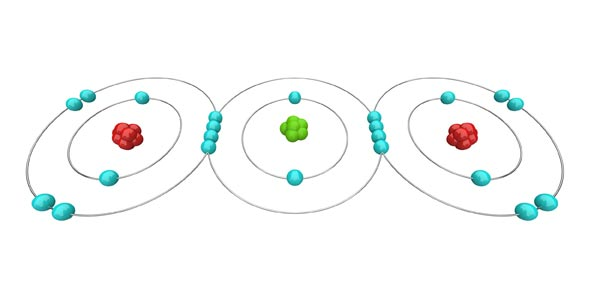# Covalent & Ionic Bonds!

10 Questions | Total Attempts: 158SettingsIonic Bonds vs Covalent Bonds

• 1.
A one-atom ion is called a(n)
• A.

Cation

• B.

Monatomic ion

• C.

Polyatomic ion

• D.

Oxyanion

• E.

Anion

• 2.
The name for the ion with fewer oxygen atoms ends with
• A.

Ite

• B.

Ate

• C.

Ide

• D.

Ion

• 3.
The force that holds two atoms together is called a
• A.

Ionic bond

• B.

Valence level

• C.

Chemical bond

• D.

Octet

• 4.
What is the family of elements that have very little tendency to react
• A.

Noble gases

• B.

Nonmetals

• C.

Metals

• D.

Ions

• 5.
The overall charge of a formula unit for an ionic compound
• A.

Is always positive

• B.

May have any value

• C.

Is always negative

• D.

Is always zero

• 6.
Ionic bonds generally occur between
• A.

A metal and a nonmetal

• B.

Metals

• C.

Nobles gases

• D.

Nonmentals

• 7.
An ionin bond is
• A.

Attraction of atoms for electrons they share

• B.

A force that holds together atoms that are oppositely charged

• C.

The movement of electrons from one atoms to another

• D.

A sea of electrons surrounding the ions

• 8.
The energy of an ionic compound is higher than that of the separate elements that formed it.
• A.

True

• B.

False

• 9.
What is the small number used to represent the number of ions of a given element in a chemical formula?
• A.

Oxidant

• B.

Cation

• C.

Subscript

• D.

Perchlorate

• 10.
The formation of a stable ionic compound from ions
• A.

Is exothermic

• B.

Is endothermic

• C.

Could be exothermic or endothermic depending

Related TopicsBack to top